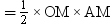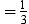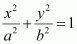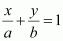Request a Free Counselling Session from our Expert Mentor# NCERT Solution for Class 12 Mathematics Chapter 8

NCERT Solution for Class 12 Mathematics Chapter 8, “Application of Integrals,” is an essential study material designed to help students understand the practical applications of integrals. Swastik Classes, a leading coaching institute, has developed comprehensive NCERT solutions that provide step-by-step explanations and solved examples to help students develop a deeper understanding of the subject. The chapter covers topics such as finding the area of a region, volumes of solids of revolution, and applications of integrals in physics and engineering. With the help of Swastik Classes’ NCERT solutions, students can improve their problem-solving skills and gain the confidence to tackle complex integral problems. These solutions are also useful for students who are preparing for competitive exams like JEE, NEET, and other entrance exams. Overall, Swastik Classes’ NCERT Solution for Class 12 Mathematics Chapter 8 is an essential resource for students who want to excel in mathematics and build a strong foundation in integral calculus.

## Answers of Mathematics NCERT solutions for class 12 Chapter 8 Application of Integrals

Exercise 8.1

Question 1:

Find the area of the region bounded by the curve y2 = x and the lines x = 1, x = 4 and the x-axis.

The area of the region bounded by the curve, y2 = x, the lines, x = 1 and x = 4, and the x-axis is the area ABCD.

Question 2:

Find the area of the region bounded by y2 = 9x, x = 2, x = 4 and the x-axis in the first quadrant.

The area of the region bounded by the curve, y2 = 9xx = 2, and x = 4, and the x-axis is the area ABCD.

Question 3:

Find the area of the region bounded by x2 = 4yy = 2, y = 4 and the y-axis in the first quadrant.

The area of the region bounded by the curve, x2 = 4yy = 2, and y = 4, and the y-axis is the area ABCD.

Question 4:

Find the area of the region bounded by the ellipse

The given equation of the ellipse, , can be represented as

It can be observed that the ellipse is symmetrical about x-axis and y-axis.

∴ Area bounded by ellipse = 4 × Area of OAB

Therefore, area bounded by the ellipse = 4 × 3π = 12π units

Question 5:

Find the area of the region bounded by the ellipse

The given equation of the ellipse can be represented as

It can be observed that the ellipse is symmetrical about x-axis and y-axis.

∴ Area bounded by ellipse = 4 × Area OAB

Therefore, area bounded by the ellipse

Question 6:

Find the area of the region in the first quadrant enclosed by x-axis, line and the circle

The area of the region bounded by the circle,  and the x-axis is the area OAB.

The point of intersection of the line and the circle in the first quadrant is

Area OAB = Area ΔOCA + Area ACB

Area of OAC  ….(i)

Area of ABC

Therefore, required area enclosed =

square units

Question 7:

Find the area of the smaller part of the circle x2 + y2 = a2 cut off by the line

The area of the smaller part of the circle, x2 + y2 = a2, cut off by the line,  is the area ABCDA.

It can be observed that the area ABCD is symmetrical about x-axis.

∴ Area ABCD = 2 × Area ABC

Therefore, the area of smaller part of the circle, x2 + y2 = a2, cut off by the line,  is  units.

*Question 8:

The area between x = y2 and x = 4 is divided into two equal parts by the line x = a, find the value of a.

The line, x = a, divides the area bounded by the parabola and x = 4 into two equal parts.

âˆ´ Area OAD = Area ABCD

It can be observed that the given area is symmetrical about x-axis.

â‡’ Area OED = Area EFCD

From (1) and (2), we obtain

Therefore, the value of a is

*Question 9:

Find the area of the region bounded by the parabola x2 and

The area bounded by the parabola, x2 = y, and the line, can be represented as

The given area is symmetrical about y-axis.

âˆ´ Area OACO = Area ODBO

The point of intersection of parabola, x2 = y, and line, x, is A (1, 1).

Area of OACO = Area of OAM – Area or OMACO

Area of Î”OAMArea of OMACO

Area of OACO = Area of OAM − Area of OMACO

Therefore, required area

*Question 10:

Find the area bounded by the curve x2 = 4y and the line x = 4– 2

The area bounded by the curve, x2 = 4y, and line, x = 4– 2, is represented by the shaded area OBAO.

Let A and B be the points of intersection of the line and parabola.

Coordinates of point A are

Coordinates of point B are (2, 1).

We draw AL and BM perpendicular to x-axis.

It can be observed that,

Area OBAO = Area OBCO + Area OACO … (1)

Then, Area OBCO = Area OMBC – Area OMBO

Similarly, Area OACO = Area OLAC – Area OLAO

Therefore, required area

*Question 11:

Find the area of the region bounded by the curve y2 = 4x and the line x = 3

The region bounded by the parabola, y2 = 4x, and the line, x = 3, is the area OACO.

The area OACO is symmetrical about x-axis.

∴ Area of OACO = 2 (Area of OAB)

Therefore, the required area is  units.

*Question 12:

Area lying in the first quadrant and bounded by the circle x2 + y2 = 4 and the lines x = 0 and = 2 is

A.

B.

C.

D.

The area bounded by the circle and the lines, x = 0 and x = 2, in the first quadrant is represented as

Thus, the correct answer is A.

*Question 13:

Area of the region bounded by the curve y2 = 4xy-axis and the line y = 3 is

A. 2

B.

C.

D.

The area bounded by the curve, y2 = 4xy-axis, and y = 3 is represented as

Thus, the correct answer is B.

Exercise 8.2

Question 1:

Find the area of the circle 4x2 + 4y2 = 9 which is interior to the parabola x2 = 4y

The required area is represented by the shaded area OBCDO.

Solving the given equation of circle, 4x2 + 4y2 = 9, and parabola, x2 = 4y, we obtain the point of intersection as and

It can be observed that the required area is symmetrical about y-axis.

∴ Area OBCDO = 2 × Area OBCO

We draw BM perpendicular to OA.

Therefore, the coordinates of M are

Therefore, Area OBCO = Area OMBCO – Area OMBO

CONFUSION

Therefore, the required area OBCDO is  units

Question 2:

Find the area bounded by curves (x – 1)2 + y2 = 1 and x2 + y 2 = 1

The area bounded by the curves, (x – 1)2 + y2 = 1 and x2 + y 2 = 1, is represented by the shaded area as

On solving the equations, (x – 1)2 + y2 = 1 and x2 + y 2 = 1, we obtain the point of intersection as A and B

It can be observed that the required area is symmetrical about x-axis.

∴ Area OBCAO = 2 × Area OCAO

We join AB, which intersects OC at M, such that AM is perpendicular to OC.

The coordinates of M are .

Therefore, required area OBCAO =

Question 3:

Find the area of the region bounded by the curves y = x+ 2, xx = 0 and x = 3

The area bounded by the curves, y = x+ 2, xx = 0, and x = 3, is represented by the shaded area OCBAO as

Then, Area OCBAO = Area ODBAO – Area ODCO

Question 4:

Using integration finds the area of the region bounded by the triangle whose vertices are (–1, 0), (1, 3) and (3, 2).

BL and CM are drawn perpendicular to x-axis.

It can be observed in the following figure that,

Area (ΔACB) = Area (ALBA) + Area (BLMCB) – Area (AMCA) … (1)

Equation of line segment AB is

Equation of line segment BC is

Equation of line segment AC is

Therefore, from equation (1), we obtain

Area (ΔABC) = (3 + 5 – 4) = 4 units

*Question 5:

Using integration find the area of the triangular region whose sides have the equations y = 2x +1, y = 3x + 1 and = 4.

The equations of sides of the triangle are y = 2x +1, y = 3x + 1, and = 4.

On solving these equations, we obtain the vertices of triangle as A(0, 1), B(4, 13), and C (4, 9).

It can be observed that,

Area (ΔACB) = Area (OLBAO) –Area (OLCAO)

*Question 6:

Smaller area enclosed by the circle x2 + y2 = 4 and the line x + y = 2 is

A.  2 (π – 2)

B.  π – 2

C.  2π – 1

D.  2 (π + 2)

The smaller area enclosed by the circle, x2 + y2 = 4, and the line, x + y = 2, is represented by the shaded area ACBA as

It can be observed that,

Area ACBA = Area OACBO – Area (ΔOAB)

Thus, the correct answer is B.

*Question 7:

Area lying between the curve y2 = 4x and y = 2x is

A.

B.

C.

D.

The area lying between the curve, y2 = 4x and y = 2x, is represented by the shaded area OBAO as

The points of intersection of these curves are O (0, 0) and A (1, 2).

We draw AC perpendicular to x-axis such that the coordinates of C are (1, 0).

Area OBAO = Area (OCABO) − Area (OCA)square units

Thus, the correct answer is B.

Miscellaneous Exercise

Question 1:

Find the area under the given curves and given lines:

(i)  y = x2x = 1, x = 2 and x-axis

(ii)  y = x4x = 1, x = 5 and x –axis

1. The required area is represented by the shaded area ADCBA as
1. The required area is represented by the shaded area ADCBA as

Question 2:

Find the area between the curves y = x and y = x2

The required area is represented by the shaded area OBAO as

The points of intersection of the curves, y = x and y = x2, is A (1, 1).

We draw AC perpendicular to x-axis.

∴ Area (OBAO) = Area (ΔOCA) – Area (OCABO) … (1)

Question 3:

Find the area of the region lying in the first quadrant and bounded by y = 4x2x = 0, y = 1 and = 4

The area in the first quadrant bounded by y = 4x2x = 0, y = 1, and = 4 is represented by the shaded area ABCDA as

Area of ABCDA = ∫14 x dy

=∫14 y2 dy    as, y = 4×2

=12∫14y dy

=12×23y3/214

=1343/2 – 13/2

=138 – 1

=13×7

=73 square units

Question 4:

Sketch the graph of  and evaluate

The given equation is

The corresponding values of and y are given in the following table.

On plotting these points, we obtain the graph of  as follows.

It is known that,

Question 5:

Find the area bounded by the curve y = sin between x = 0 and x = 2π

The graph of y = sin x can be drawn as

∴ Required area = Area OABO + Area BCDB

Question 6:

Find the area enclosed between the parabola y2 = 4ax and the line y mx

The area enclosed between the parabola, y2 = 4ax, and the line, y mx, is represented by the shaded area OABO as

The points of intersection of both the curves are (0, 0) and

We draw AC perpendicular to x-axis.

∴ Area OABO = Area OCABO – Area (ΔOCA)

Question 7:

Find the area enclosed by the parabola 4y = 3x2 and the line 2y = 3x + 12

The area enclosed between the parabola, 4y = 3x2, and the line, 2y = 3x + 12, is represented by the shaded area OBAO as

The points of intersection of the given curves are A (–2, 3) and (4, 12).

We draw AC and BD perpendicular to x-axis.

∴ Area OBAO = Area CDBA – (Area ODBO + Area OACO)

Question 8:

Find the area of the smaller region bounded by the ellipse  and the line

The area of the smaller region bounded by the ellipse, , and the line, , is represented by the shaded region BCAB as

∴ Area BCAB = Area (OBCAO) – Area (OBAO)

Question 9:

Find the area of the smaller region bounded by the ellipse   and the line

The area of the smaller region bounded by the ellipse,, and the line,, is represented by the shaded region BCAB as

∴ Area BCAB = Area (OBCAO) – Area (OBAO)

Question 10:

Find the area of the region enclosed by the parabola x2 = y, the line y = x + 2 and x-axis

The area of the region enclosed by the parabola, x2 = y, the line, y = x + 2, and x-axis is represented by the shaded region OACO as

The point of intersection of the parabola, x2 = y, and the line, y = x + 2, is A (–1, 1) and C(2, 4).

Area of

*Question 11:

Using the method of integration find the area bounded by the curve

[Hint: the required region is bounded by lines x + y = 1, x – y = 1, – x + y = 1 and – x – = 11]

The area bounded by the curve,  is represented by the shaded region ADCB as

The curve intersects the axes at points A (0, 1), B (1, 0), C (0, –1), and D (–1, 0).

It can be observed that the given curve is symmetrical about x-axis and y-axis.

∴ Area ADCB = 4 × Area OBAO

*Question 12:

Find the area bounded by curves

The area bounded by the curves, , is represented by the shaded region as

It can be observed that the required area is symmetrical about y-axis.

*Question 13:

Using the method of integration find the area of the triangle ABC, coordinates of whose vertices are A (2, 0), B (4, 5) and C (6, 3)

The vertices of ΔABC are A (2, 0), B (4, 5), and C (6, 3).

Equation of line segment AB is

Equation of line segment BC is

Equation of line segment CA is

Area (ΔABC) = Area (ABLA) + Area (BLMCB) – Area (ACMA)

*Question 14:

Using the method of integration find the area of the region bounded by lines:

2x + y = 4, 3x – 2y = 6 and x – 3+ 5 = 0

The given equations of lines are

2x + y = 4 … (1)

3x – 2y = 6 … (2)

And, x – 3+ 5 = 0 … (3)

The area of the region bounded by the lines is the area of ΔABC. AL and CM are the perpendiculars on x-axis.

Area (ΔABC) = Area (ALMCA) – Area (ALB) – Area (CMB)

*Question 15:

Find the area of the region

The area bounded by the curves, , is represented as

The points of intersection of both the curves are

The required area is given by OABCO.

It can be observed that area OABCO is symmetrical about x-axis.

∴ Area OABCO = 2 × Area OBC

Area OBCO = Area OMC + Area MBC

Therefore, the required area is  units

*Question 16:

Area bounded by the curve y = x3, the x-axis and the ordinates x = –2 and x = 1 is

A.  B.  C.  D.

Required Area =

Thus, the correct answer is D.

*Question 17:

The area bounded by the curve  x-axis and the ordinates x = –1 and x = 1 is given by

[Hint: y = x2 if x > 0 and y = –x2 if x < 0]

A. 0 B.  C.  D.

Thus, the correct answer is C.

*Question 18:

The area of the circle x2 + y2 = 16 exterior to the parabola y2 = 6x is

A.  B.  C.  D.

The given equations are

x2 + y2 = 16       … (1)

y2 = 6x               … (2)

Area bounded by the circle and parabola

= 2 [Area of OACO + Area of ACBA]

Thus, required area

Thus, the correct answer is C.

*Question 19:

The area bounded by the y-axis, y = cos x and y = sin x when

A.  B.  C.  D.

The given equations are

y = cos x … (1)

And, y = sin x … (2)

Required area = Area (ABLA) + area (OBLO)

Integrating by parts, we obtain

Thus, the correct answer is B.

## Conclusion

Swastik Classes’ NCERT Solution for Class 12 Mathematics Chapter 8, “Application of Integrals,” provides comprehensive study material that helps students understand the practical applications of integrals. The solutions offer step-by-step explanations and solved examples that help students develop a deeper understanding of the subject. The chapter covers various topics, including finding the area of a region, volumes of solids of revolution, and applications of integrals in physics and engineering. With the help of these solutions, students can improve their problem-solving skills and gain the confidence to tackle complex integral problems. Swastik Classes’ NCERT solutions are designed according to the latest CBSE syllabus, making them useful for students preparing for board exams or competitive exams like JEE and NEET. Overall, Swastik Classes’ NCERT Solution for Class 12 Mathematics Chapter 8 is an excellent resource for students who want to excel in mathematics and build a strong foundation in integral calculus.

Multiple exercises are covered in Chapter 8 – Application of Integrals. For a better understanding, the answer to each question in each exercise is offered along with detailed, step-by-step answers.

### Why opt for SWC?

One of the top IIT JEE coaching institutes is Swastik Classes. Shobhit Bhaiya and Alok Bhaiya, pioneering mentors of IIT JEE Coaching Classes, started Swastik Classes in Anand Vihar. Over the last 15 years, they have educated and sent over 2000+ students to IITs and 5000+ students to different famous universities such as BITS, NITs, DTU, and NSIT. When it comes to coaching programmes for IIT JEE, Swastik Classes is the top IIT JEE Coaching in Delhi, favoured by students from all over India.

Swastik Classes’ teachers have a solid academic background, having graduated from IIT with honours, and have extensive expertise in moulding students’ careers.

The study process in Swastik courses is separated between pre-class and post-class work, which is one of the most significant aspects. They are precisely created to improve the student’s mental ability and comprehension.

## Frequently Asked Questions on NCERT Solutions Class 12 Maths Chapter 8 – Application of Integrals

### How do you solve an integral application?

Functions, differentiation, and integration are only a few examples of calculating procedures. Integrals are used in a variety of domains, including mathematics, science, and engineering. In addition, we mostly employ integral formulae to calculate areas or irregular forms in a two-dimensional space.

### What is the difference between integration and differentiation?

Differentiation is a technique for analysing tiny changes in one quantity in relation to a unit change in another. Integration, on the other hand, is used to combine little and discrete data that cannot be combined separately and represented in a single value.

### Which chapter is important in 12th maths?

Almost all the chapters are important for maths CBSE class 12 but if i would talk about 1 most important chapter then it would be INTEGRALS because it has the most weightage in 12th exam.

### How many exercises are in application of integration?

2